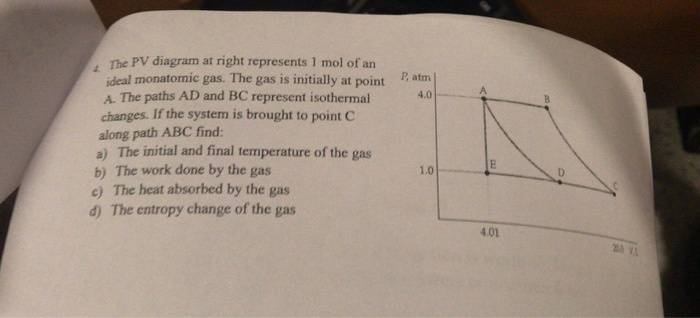# Thermodyanamics PV diagram

• Muthumanimaran

## Homework Statement## Homework Equations

Ideal gas law:
$$PV=nRT$$

Workdone by the gas:
For Isobaric process
$$W=P(V_{2}-V_{1})$$

For Isothermal Process
$$W=nRT \ln(V_{2}{/}V_{1})$$

First law:
$$U=Q-W$$

## The Attempt at a Solution

subpart (a) is trivial, all we have to do is to substitute the given data in Ideal gas law to get the temperatures.

I have conceptual difficulty in part (b) and (c)only
The PV diagram conists of two Isothersms (DA and BC) and two Isobars (AB and CD)
How to calculate workdone by the gas?
Is it just the sum of the workdone by four processes ?

Next, Heat absorbed by gas?
Since the total Internal energy U of a cyclic process is zero, is it just equal to the total workdone?

#### Attachments

Last edited:
How to calculate workdone by the gas?
Is it just the sum of the workdone by four processes ?
No. You are told that the system goes from A to C along path ABC. Which processes does that involve?
Next, Heat absorbed by gas?
Since the total Internal energy U of a cyclic process is zero, is it just equal to the total workdone?
Is this a cyclic process?
(By the way, your statement is wrong in any case. The total internal energy change, ΔU, of a cyclic process is zero. It is vitally important to distinguish between thermodynamic quantities and changes in those quantities. Many mistakes are made here.)

•Muthumanimaran
I think the focus of this problem is only the portion of the path between A and C. From the information given, you also know the volume at B. What is that volume?

•Muthumanimaran
Oh, I got it now. I just need to calculate the work done from A to B and B to C and sum it up? Am I right?

Oh, I got it now. I just need to calculate the work done from A to B and B to C and sum it up? Am I right?
That's my interpretation of the problem statement. Arguably, it is not very clear.

From A to C it is not a cyclic process, so the change in internal energy cannot be zero (from A to C), then how to find the heat absorbed by the gas?

What is the pressure, volume, and temperature of the gas at C? What is the pressure, volume, and temperature of the gas at B?

P=1 atm V=20lit at point C and T=PV/R

So T=20/R K at point C

Pressure at B is 4 atm T = 20/R
So V=5 lit

Without knowing the specific heat of the gas how do I calculate the change in internal energy from A to B? Workdone = P(VB-VA), is it just enough to plug these values to find the heat transfer from A to B? for B to C is an isotherm, so workdone is equal to heat right?

P=1 atm V=20lit at point C and T=PV/R

So T=20/R K at point C

Pressure at B is 4 atm T = 20/R
So V=5 lit
You do know the value of R, correct.? Please provide actual numbers for the temperatures at A, B, and C.

Without knowing the specific heat of the gas how do I calculate the change in internal energy from A to B? Workdone = P(VB-VA), is it just enough to plug these values to find the heat transfer from A to B? for B to C is an isotherm, so workdone is equal to heat right?
You are told that the gas is an ideal monoatomic gas. What does that tell you about its specific heat?

You are told that the gas is an ideal monoatomic gas. What does that tell you about its specific heat?
Specific heat is (3/2)R. Now I got it.